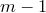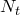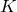Want to share your content on R-bloggers? click here if you have a blog, or here if you don't.

I am often asked how to fit an ARIMA or ETS model with data having a long seasonal period such as 365 for daily data or 48 for half-hourly data. Generally, seasonal versions of ARIMA and ETS models are designed for shorter periods such as 12 for monthly data or 4 for quarterly data.

The `ets()` function in the forecast package restricts seasonality to be a maximum period of 24 to allow hourly data but not data with a larger seasonal frequency. The problem is that there areparameters to be estimated for the initial seasonal states whereis the seasonal period. So for large, the estimation becomes almost impossible.

The `arima()` function will allow a seasonal period up tobut in practice will usually run out of memory whenever the seasonal period is more than about 200. I haven’t dug into the code to find out why this is the case — theoretically it would be possible to have any length of seasonality as the number of parameters to be estimated does not depend on the seasonal order. However, seasonal differencing of very high order does not make a lot of sense — for daily data it involves comparing what happened today with what happened exactly a year ago and there is no constraint that the seasonal pattern is smooth.

For such data I prefer a Fourier series approach where the seasonal pattern is modelled using Fourier terms with short-term time series dynamics allowed in the error. For example, consider the following model:whereis an ARIMA process. The value ofcan be chosen by minimizing the AIC.

This is easily fitted using R. Here is an example with,andan ARIMA(2,0,1) process:

```n <- 2000
m <- 200
y <- ts(rnorm(n) + (1:n)%%100/30, f=m)
fourier <- function(t,terms,period)
{
n <- length(t)
X <- matrix(,nrow=n,ncol=2*terms)
for(i in 1:terms)
{
X[,2*i-1] <- sin(2*pi*i*t/period)
X[,2*i] <- cos(2*pi*i*t/period)
}
colnames(X) <- paste(c("S","C"),rep(1:terms,rep(2,terms)),sep="")
return(X)
}

library(forecast)
fit <- Arima(y, order=c(2,0,1), xreg=fourier(1:n,4,m))
plot(forecast(fit, h=2*m, xreg=fourier(n+1:(2*m),4,m)))```

The advantages of this approach are:

• it allows any length seasonality;
• for data with more than one seasonal period, you can include Fourier terms of different frequencies;
• the seasonal pattern is smooth for small values of(but more wiggly seasonality can be handled by increasing);
• the short-term dynamics are easily handled with a simple ARMA error.

The only real disadvantage (compared to a seasonal ARIMA model) that I can think of is that the seasonality is assumed to be fixed — the pattern is not allowed to change over time. But in practice, seasonality is usually remarkably constant so this is not a big disadvantage except for very long time series.

The order ofcan also be chosen automatically:

`fit <- auto.arima(y, D=0, max.P=0, max.Q=0, xreg=fourier(1:n,4,m))`

Note that the seasonal parts of the ARIMA model should all be set to zero as we do not wantto be a seasonal ARIMA model.

I’ll add the `fourier()` function to the forecast package on the next release.

It is much harder to do something like this for ETS models. One of our students has been working on this and our paper on complex seasonality describes the procedure. We plan to add this functionality to the forecast package soon, but the code is not quite ready yet.